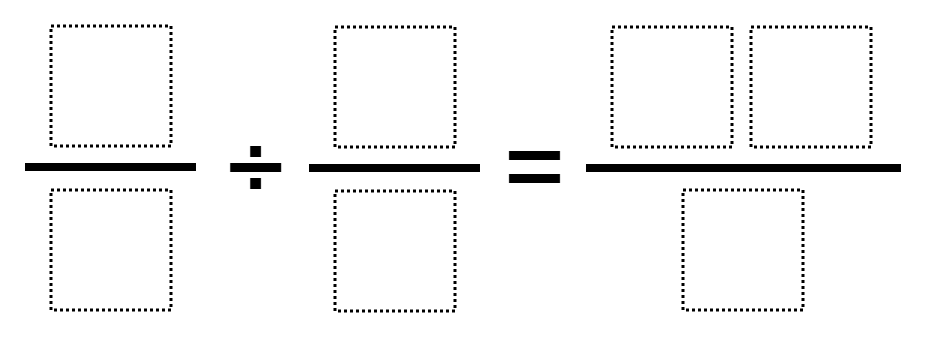Home > Grade 6 > Fraction Division

# Fraction Division

Directions: Use the digits 0 through 9, without repeats, to solve the problem below.### Hint

How do you divide by a fraction?

Source: Shaun Errichiello

## Largest Possible GCF #2

Directions: Using the digits 0-9 at most once, fill in the boxes to make the …

1.I think these are all the possibilities, listed here in form a/b divided by c/d, but where a and d can be switched and/or b and c can be switched:

5/2 divided by 4/6 = 30/8
5/2 divided by 3/8 = 40/6 [as above]
7/2 divided by 4/9 = 63/8

2.Would this a possible solution also? Or would it be invalid?

9/3 divided by 1/6 equals 72/4

•I think 9/3 divided by 1/6 would be 72/3. I think it might be cool to have a class of solutions like this, where there is 1 repeated digit. In this case, the 1.

•9/3 divided by 1/6 would actually be 54/3 so it wouldn’t work since the “3” is repeated.

•“9/3 divided by 1/6 equals 72/4” was original query

9/3 divided by 1/6 reduces to 54/3 hence 18
72/4 reduces to 18

Hence 18=18

I suppose the question is of repeats in original equation or no repeats in all the equations as you simplify and reduce.

3.My student found this solution:
9/2 divided by 4/7 equals 63/8